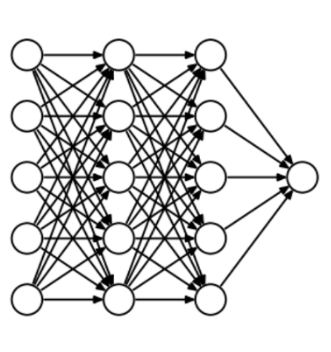/  Deep Learning Interview questions and answers   /  Explain Recurrent Neural Network?## Explain Recurrent Neural Network?

Ans: Recurrent Neural Network are a type of Neural Network where the output from previous step are fed as input to the current step. In traditional neural networks, all the inputs and outputs are independent to each other, but when it is required to predict the next word of a sentence, the previous words are required and hence there is a requirement to remember the previous words. RNN solved this issue with the help of a Hidden Layer. The foremost important feature of RNN is Hidden state, which remembers some information about a sequence.RNN have a memory which remembers all information about the calculations. It uses the same parameters for each input as it performs the same task on all the inputs or hidden layers to produce the output. This decreases the complexity of parameters, unlike other neural networks.

RNN converts the independent activations into dependent activations by providing the same weights and biases to all the layers, thus reducing the complexity of increasing parameters and memorizing each previous output by giving each output as input to the next hidden layer.

Hence these three layers can be joined together such that the weights and bias of all the hidden layers is the same, into a single recurrent layer.Formula for calculating current state:where:

ht -> current state

ht-1 -> previous state

xt -> input state

Formula for applying Activation function(tanh):where:

whh -> weight at recurrent neuron

wxh -> weight at input neuron

Formula for calculating output:Yt -> output

Why -> weight at output layer

Training through RNN

A single time step of the input is provided to the Recurrent Neural Network.

Calculate its current state using a set of current input and the previous state.

The current ht becomes ht-1 for the next time step.

Number of iterations can take place in every step in the problem and join the information from all the previous states.

Once all the time steps are completed the final current state is used to calculate the output.

The output is then compared to the target output and the error is generated.

The error is then back-propagated to the network which updates the weights and hence the network (RNN) is trained.